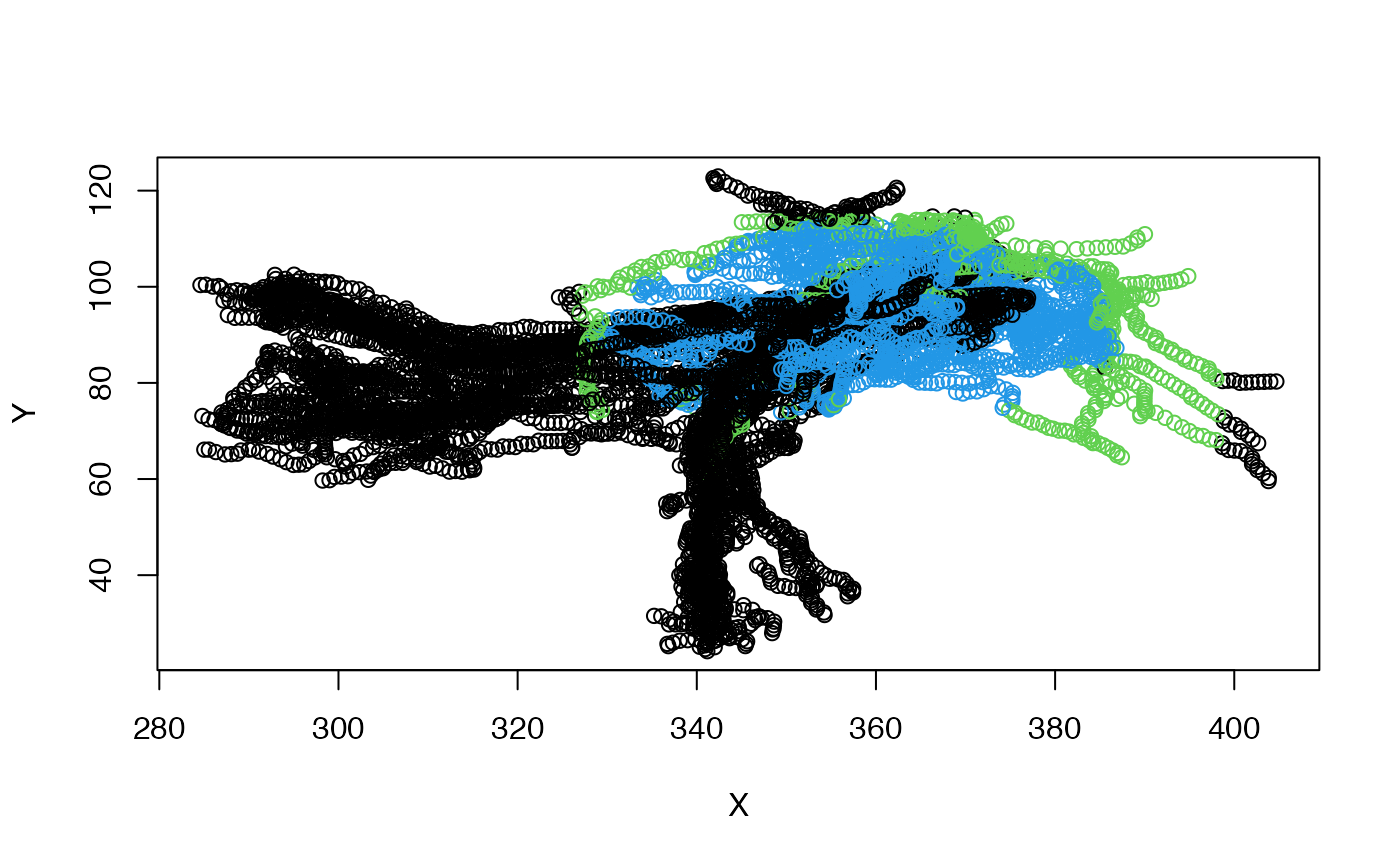Find which points of an object are inside a surface

pointsinside(x, surf, ...)

# S3 method for default
pointsinside(
x,
surf,
...,
rval = c("logical", "distance", "mesh3d", "consistent_logical")
)

## Arguments

x an object with 3D points. The reference surface - either a mesh3d object or any object that can be converted using as.mesh3d including hxsurf and ashape3d objects. additional arguments for methods, eventually passed to as.mesh3d. what to return.

## Value

A vector of logical values or distances (positive inside, negative outside) equal to the number of points in x or the mesh3d object returned by Rvcg::vcgClostKD.

## Details

Note that hxsurf surface objects will be converted to mesh3d before being passed to Rvcg::vcgClostKD, so if you are testing repeatedly against the same surface, it may make sense to pre-convert.

pointsinside depends on the face normals for each face pointing out of the object (see example). The face normals are defined by the order of the three vertices making up a triangular face. You can flip the face normal for a face by permuting the vertices (i.e. 1,2,3 -> 1,3,2). If you find for a given surface that points are outside when you expect them to be inside then the face normals are probably all the wrong way round. You can invert them yourself or use the Morpho::invertFaces function to fix this.

The rval argument determines the return value. These options should be fairly clear, but the difference between logical and consistent_logical needs some explanation. The logical method now does a pre-test to remove any points that are not in the 3D bounding box (cuboid) enclosing the surf object. This often results in a significant speed-up by rejecting distant points and has the additional benefit of rejecting distant points that sometimes are erroneously declared inside the mesh (see below). Regrettably it is not yet possible to extend this approach when distances are being returned, which means there will be a discrepancy between the results of rval="logical" and looking for points with distance >=0. If you want to ensure consistency between these approaches, use rval="consistent_logical".

If you find that some points but not all points are not behaving as you would expect, then it may be that some faces are not coherently oriented. The Rvcg::vcgClean function can sometimes be used to correct the orientation of the faces. Fixing more problematic cases may be possible by generating a new surface using alphashape3d::ashape3d (see examples).

## Examples

# check if the vertices in these neurons are inside the mushroom body calyx
# surface object
inout=pointsinside(kcs20, surf=subset(MBL.surf, "MB_CA_L"))
table(inout)
#> inout
#> FALSE  TRUE
#>  6530  1991 # you can also check if points are inside a bounding box
mbcalbb=boundingbox(subset(MBL.surf, "MB_CA_L"))
inout2=pointsinside(kcs20, mbcalbb)
# compare those two
table(inout, inout2)
#>        inout2
#> inout   FALSE TRUE
#>   FALSE  5691  839
#>   TRUE      0 1991pts=xyzmatrix(kcs20)
# nb that colour expressions maps combinations of two logicals onto 1:4
plot(pts[,1:2], col=1+inout+inout2*2)# the colours are defined by
palette()[1:4]
#>  "black"   "#DF536B" "#61D04F" "#2297E6"
# be a bit more lenient and include points less than 5 microns from surface
MBCAL=subset(MBL.surf, "MB_CA_L")
inout5=pointsinside(kcs20, surf=MBCAL, rval='distance') > -5
table(inout5)
#> inout5
#> FALSE  TRUE
#>  5604  2917 # \donttest{
# show which points are in or out
# Hmm seems like there are a few red points in the vertical lobe
# that are well outside the calyx
points3d(xyzmatrix(kcs20), col=ifelse(inout5, 'red', 'black'))
plot3d(MBL.surf, alpha=.3)

# Let's try to make an alphashape for the mesh to clean it up
library(alphashape3d)
MBCAL.as=ashape3d(xyzmatrix(MBCAL), alpha = 10)
# Plotting the points, we can see that is much better behaved
points3d(xyzmatrix(kcs20),
col=ifelse(pointsinside(kcs20, MBCAL.as), 'red', 'black'))
# }

if (FALSE) {
# Show the face normals for a surface
if(require('Morpho')) {
# convert to a mesh3d object used by rgl and Morpho packge
MBCAL.mesh=as.mesh3d(subset(MBL.surf, "MB_CA_L"))
fn=facenormals(MBCAL.mesh)
wire3d(MBCAL.mesh)
# show that the normals point out of the object
plotNormals(fn, long=5, col='red')

# invert the faces of the mesh and show that normals point in
MBCAL.inv=invertFaces(MBCAL.mesh)
plotNormals(facenormals(MBCAL.inv), long=5, col='cyan')
}
}